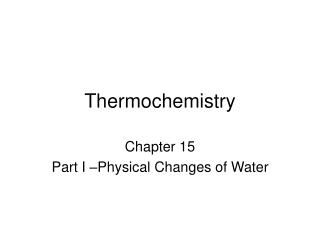DownloadDownload PresentationThermochemistry

# Thermochemistry

Télécharger la présentation## Thermochemistry

- - - - - - - - - - - - - - - - - - - - - - - - - - - E N D - - - - - - - - - - - - - - - - - - - - - - - - - - -
##### Presentation Transcript

1. Thermochemistry Chapter 15 Part I –Physical Changes of Water

2. Factors that influence heat change (q).

3. Heating curve: Ice  Water  Steam (H2O @ 1 atm) Temperature Energy Added

4. Critical Information 4.18 J = 1 cal Dq = mcDT (same phase energy change) Dq = mDh (phase change) cice = 2.1 J/goC cwater = 4.18 J/goC = 1.00 cal/goC csteam = 1.7 J/goC Dhfus = 334 J/g Dhvap = 2261 J/g

5. Heating Curve Problems You should be able to solve for: q total:

6. q total problem How much heat needs to be removed from 84 g of steam at 412 K to turn it into ice at 218 K?

7. Heating Curve Problems You should be able to solve for: Final temperature:

8. Tf problem What is the final temperature if 78 kJ of heat are added to 22 g of ice at -14oC?

9. Heating Curve Problems You should be able to solve for: % sample in two different phases:

10. % sample in two different phases problem How many grams of each phase will be present if 50 kJ of heat are added to 22 g of ice at -14oC?

11. Heating Curve Problems You should be able to solve for: Mass:

12. Mass problem What is the mass of a sample of H2O if the addition of 35 kJ of heat takes it from 250 K to 415 K?

13. Heating Curve Problems You should be able to solve for: q total: Add the energy for each step on the graph Final temperature: Subtract the energy for each step from the total energy until the energy runs out during a step. Use the energy remaining for that step to solve for DT, TF. % sample in two different phases: Subtract the energy for each step from the total energy until the energy runs out during a plateau. Divide the energy remaining for that step by the total energy needed to change phase completely. Multiply the mass by this fraction. Mass –factor out m from each step.

14. Practice Problem A sample of ice at -25oC is heated to steam at 200oC by the addition of 300 kJ of energy. What is the mass of the sample?